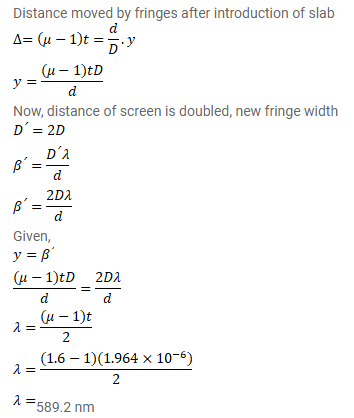# In a Young's double slit experiment using monochromatic light,

Question:

In a Young's double slit experiment using monochromatic light, the fringe pattern shifts by a certain distance on the screen when a mica sheet of refractive index $1.6$ and thickness $1.964$ micron ( 1 micron $=10^{-5} \mathrm{~m}$ is introduced in the path of one of the interfering waves. The mica sheet is then removed and the distance between the screen and slits is doubled. It is found that the distance between the successive maxima now is the same as the observed fringe-shift upon the introduction of the mica sheet. Calculate the wavelength of the monochromatic light used in the experiment.

Solution: